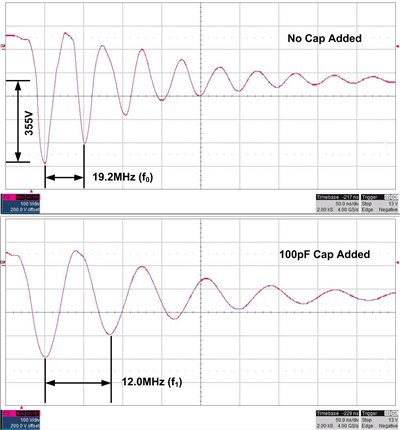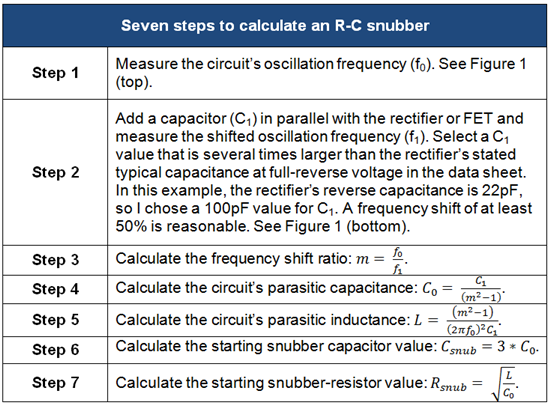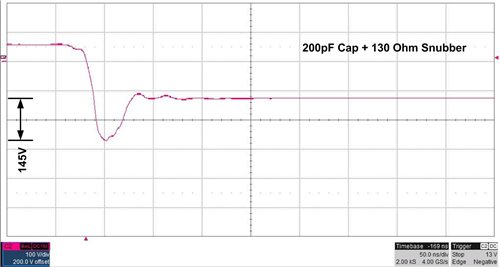# Power Tips: Calculate an R-C snubber in seven steps

Ringing in switching power supplies can generate radiated and conducted noise, create circuit jitter and excessive dissipation, and easily overstress components. Ringing is a major concern in applications such as audio, processor power and any design that requires electromagnetic interference (EMI) qualification.

Often, you can tame the circuit by adding a simple resistor-capacitor (R-C) snubber to “damp out the ringing.” In this post, I’ll outline a seven-step procedure that can help take the guesswork out of selecting your snubber values.

Ringing can occur in any switching converter where an inductance and a capacitance form an inductor-capacitor (L-C) tank. The inductance may be from component leads, printed circuit board traces and transformer leakage, while the capacitance may be due to nonlinear components such as rectifiers and interwinding transformer capacitance. The ring frequency and amplitude are generally unknown since most circuit parasitics are typically unknown. Two common locations where excessive ringing occurs (at least in converters such as a flyback) are across the rectifier diode and the switching FET. One simple solution to reduce this ringing is by damping or “snubbing” it with a series R-C circuit, typically placed directly across the rectifier or FET.

The seven-step procedure shown in Table 1 uses a common methodology that shifts the resonant frequency of the ringing to calculate the circuit’s parasitic capacitance (Co) and inductance (L). Once you know those, you can calculate the snubber capacitor (Csnub) and resistor (Rsnub). I took the example waveforms in Figures 1 and 2 with an R-C snubber placed in parallel with the rectifier in the 9Vdc-57Vdc Input, 56V/20W Isolated Flyback Reference Design, but the procedure is the same if used across the FET.Figure 1: Unsnubbed rectifier ringing (top) and frequency-shifted ringing (bottom)Figure 2 shows the spike reduction and damping effect of the calculated values. You can adjust the ringing higher or lower by varying the Csnub value. Larger values for Csnub reduce the voltage-spike amplitude further, but increase power loss in Rsnub.

Alternatively, you can decrease the power dissipation in Rsnub by lowering Csnub, but ringing will increase. You must weigh the trade-off between acceptable voltage-ring amplitude and Rsnub losses.Undamped ringing in switching converters can create excessive EMI and overstress components. A properly calculated R-C snubber can be instrumental in taming these issues. I hope you’ll find my seven-step procedure simple to follow and a good starting point to help you damp out the ringing. For more about the topic covered here, see my EETimes Power Tips post “Calculating an R-C snubber.”

• Here is a useful Excel tool for making Snubber Calculations.

Snubber_Calculations.xlsx

• To be effective, the snubber capacitor needs to have sufficient capacitance at the full switching node voltage level to effectively absorb the energy stored in the parasitic inductance while charging the switching node.  In order to minimize capacitance loss due to DC bias derating, it is generally advisable to have a snubber rated for at least 2x the expected switch-node voltage level.

For a BUCK converter, that is the input voltage

For a BOOST converter, that is the output voltage

For other converter topologies, you'll want to refer to the switch node voltage of that topology.

Using a lower voltage rated capacitor will result in the capacitor absorbing less energy and thus being less effective at reducing ringing.

• I think P=1/2*c*vpk^2xFsw

• I would agree that the inductance may be from component leads, printed circuit board traces and transformer leakage, while stump removal the capacitance may be due to nonlinear components such as rectifiers and interwinding transformer capacitance.

• what should the snubber capacitor's voltage rating be?